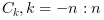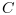Scilab Home page | Wiki | Bug tracker | Forge | Mailing list archives | ATOMS | File exchange
Change language to: English - Português - 日本語 - Русский

See the recommended documentation of this function

Aide de Scilab >> Traitement du Signal > xcorr

# xcorr

Computes discrete auto or cross correlation

### Calling Sequence

```[c [,lagindex]] = xcorr(x [,maxlags [,scaling]])
[c [,lagindex]] = xcorr(x,y [,maxlags [,scaling]])```

### Parameters

x

a vector of real or complex floating point numbers.

y

a vector of real or complex floating point numbers. The default value is `x`.

maxlags

a scalar with integer value greater than 1. The default value is `n`. Where `n` is the maximum of the `x` and `y` vector length.

scaling

a character string with possible value: `"biased"`, `"unbiased"`, `"coeff"`, `"none"`. The default value is `"none"`.

c

a vector of real or complex floating point numbers with same orientation as `x`.

lagindex

a row vector, containing the lags index corresponding to the `c` values.

### Description

• `c=xcorr(x)` computes the un-normalized discrete auto correlation:and return in `c` the sequence of auto correlation lagswith `n` is the length of `x`
• `xcorr(x,y)` computes the un-normalized discrete cross correlation:and return in `c` the sequence of auto correlation lagswith `n` is the maximum of `x` and `y` length's.

If the `maxlags` argument is given `xcorr` returns in `c` the sequence of auto correlation lags. If `maxlags` is greater than `length(x)`, the first and last values of `c` are zero.

The `scaling` argument decribes howis normalized before being returned in `c`:

• "biased":`c=``/n`.
• "unbiased":`c=``./(n-(-maxlags:maxlags))`.
• "coeff":`c=``/(norm(x)*norm(y))`.

### Remark

The corr function computes the "biased" covariance of `x` and `y` and only return in `c` the sequence of auto correlation lags.

### Method

This function computesusing `ifft(fft(x).*conj(fft(y)))`.

### Examples

```t = linspace(0, 100, 2000);
y = 0.8 * sin(t) + 0.8 * sin(2 * t);
[c, ind] = xcorr(y, "biased");
plot(ind, c)```### Authors

• Serge Steer, INRIA

fft